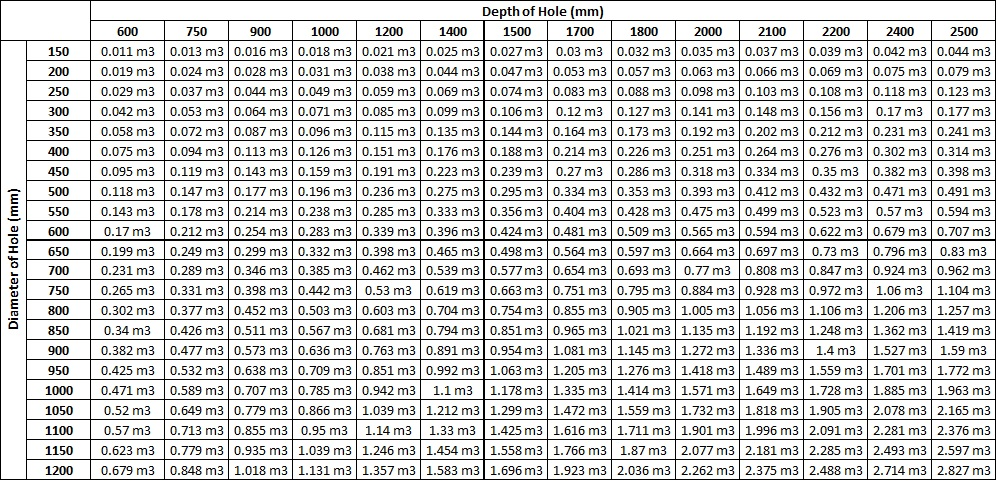# Concrete Volume Calculators

How much concrete will you need for your job? Good question. We have taken all the hard work out of this by providing the following tools below. If you have a round hole such as post holes or pillars then use the round hole table. If you want to calculate the volume of concrete required for a rectangular slab, use the rectangular slab calculator.

## To find cubic metres needed to fill a round holeEXAMPLE: How much concrete is needed to fill 5 holes 1200mm deep and 1050mm round?

ANSWER: One hole takes 1.039 cubic metres, therefore 5 holes = 5 x 1.039 = 5.195. (say 5.2 cubic metres)

NOTE: When the Diameter of a hole is doubled, it takes 4 times more concrete.

## Rectangular Slab Calculator

Volume (in m3) = Width of Slab (in m) x Length of Slab (in m) x Depth of slab (in m)

## Volume (m3): 0.00

Call the team at Ducats
02 6772 7255
148 Miller St, Armidale, 2350